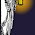## Thursday, January 30, 2014

### 13: Strange Triangles

Triangle ABC is congruent to triangle DEF.
In triangle ABC, side AB measures 9, side BC measures 3x+18, and side CA measures 7.
In triangle DEF, side DE measures 9, side EF measures 2x+26, and side FD measures 7.

What equation would help you to solve for the side length of BC and EF?

(Actual question in an on-line geometry course.)

What would you do to fix this question?

1.What reasoning could you use to determine the value of x.?

I still don't think that's a great questions, but I think it's better than the one given.
CPCTC

2.Try it and look at the values ...

3.While solving for x and finding the length of the side is straight forward enough, the resulting answer contradicts the triangle inequality. So triangle ABC and triangle DEF can't actually be triangles. The easy fix would be to change the lengths of the other sides so that the answer of 42 for side BC would make sense.

4.What would you do to fix this question?

Clearly, changing the values of x so the triangle is possible would do it, but I really don't know what this problem is supposed to be addressing in the first place. It just seems like a lazy way to get kids to solve an equation without making them think about the context of the question. It might just as well read "Maria and Desmond enjoy the rock band Modest Mouse an equal amount. If 3x+10 represents the amount of enjoyment Maria derives from listening to the band, and 2x+29 represents the amount of enjoyment Desmond gets from the band, find the value of x"

Totally meaningless.

Maybe the way to improve the question, if you really like it, is to set it in terms of similar triangles which have some scale factor other than 1, and spend the time to make sure your rules for creating the parameters of the question actually work.

For example:
Triangle ABC is similar to triangle DEF.
In triangle ABC, side AB measures 9, side BC measures 2x+4, and side CA measures 7.
In triangle DEF, side DE measures 22.5, side EF measures 7x+6, and side FD measures 17.5.